### Periodic Classification Of Element Class 10th Science And Technology Part 1 MHB Solution

Periodic Classification Of Element

Class 10th Science And Technology Part 1 MHB Solution

# Exercise

###### Exercise
Question 1.

Rearrange the columns 2 and 3 so as to match with the column 1.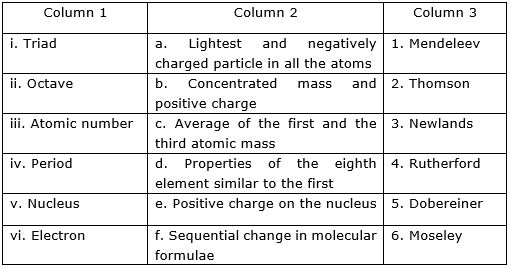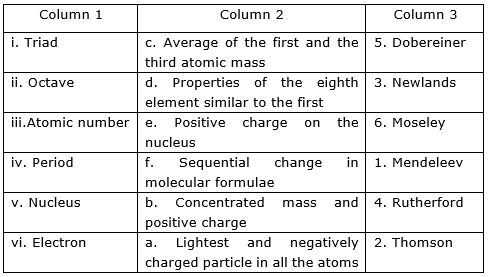Explanation:

i. The German Chemist, Johann Dobereiner was the first to consider the idea of trends among properties of elements. He made group of three elements each which are having similar properties and called them triads. He showed that the atomic mass of the middle element was approx. equal to the mean of atomic masses of other two elements. For example: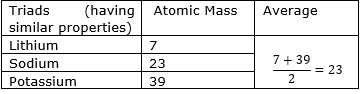ii. The English Chemist, John Newlands discovered the Law of Octaves. He arranged the elements in increasing order of their atomic masses. He noticed that every eighth element had properties similar to the first element.

iii. The English Scientist, Henry Moseley showed that the atomic number is equal to the positive charge on the nucleus or the total number of protons in the nucleus of the atom of that element.

The atomic number is represented by ‘Z’.

iv. Mendeleev organized the periodic table on the basis of chemical and physical properties of the elements. The horizontal rows are called periods. He arranged the elements sequentially in periods according to the change in molecular formula.

v. A scientist Rutherford bombarded a very thin layer of gold with alpha-particles. On the basis of observations, he concluded that:

(a) The nucleus is at the center of the atoms.

(b) It has positive charge.

(c) The positive charge and most of the mass of the atom is concentrated in the nucleus.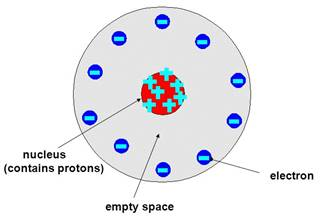vi. J.J Thomson founded that an atom has a spherical shape.

He compared an atom to a watermelon. He showed that the electrons (negatively charged particles) are embedded in it.The atom has a positively charged part.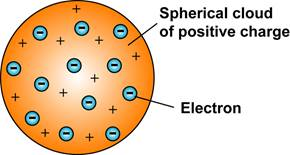Question 2.

Choose the correct option and rewrite the statement.

a. The number of electrons in the outermost shell of alkali metals is......

(i) 1 (ii) 2 (iii) 3 (iv) 7

b. Alkaline earth metals have valency 2. This means that their position in the modern periodic table is in .....

(i) Group 2 (ii) Group16

(iii) Period 2 (iv) d-block

c. Molecular formula of the chloride of an element X is XCl. This compound is a solid having high melting point. Which of the following elements be present in the same group as X.

(i) Na (ii) Mg (iii) Al (iv) Si

d. In which block of the modern periodic table are the nonmetals found?

(i) s-block (ii) p-block

(iii) d-block (iv) f-block

a) The number of electrons in the outermost shell of alkali metals is 1.

Explanation:

Alkali metals belong to the group first of the periodic table. The number of electrons in the outermost shell (valence electrons) of all the alkali metals is 1 as shown below: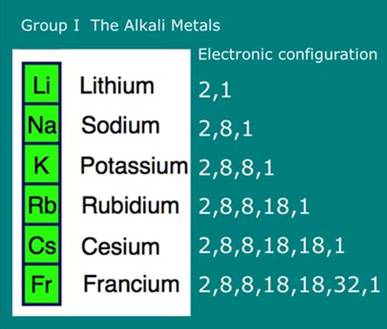b) Alkaline earth metals have valency 2. This means that their position in the modern periodic table is in Group 2.

Explanation:

It is the electronic configuration of the elements which decides the group in which they are to be placed. The alkaline earth metals have two electrons in the outermost shell. This means that their position in the periodic table is in group 2.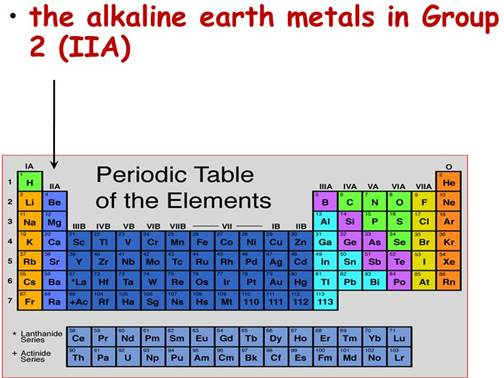Note: Count the number of electrons present in the outermost shell of any element then automatically it will tell you about the group no. of that element.

c) (i)-Na

Explanation:

From the formula XCl, we can see that one atom of X combines with one atom of chlorine(Cl). This means chlorine is combined with the element X who is having valency of one.

In the given elements, Na has one electron in the outermost shell. Hence, Na is the element X as NaCl.

d. (ii)- p-block

Explanation:

The nonmetals are found in the p-block of the periodic table.

The p- block consists of:

(a) Metals

(b) Non-metals

(c) Metalloids (behave as both metals and non-metals)

In p- block, it is seen that the zig- zag line separates the metals from nonmetals in the periodic table.

Question 3.

An element has its electron configuration as 2,8,2. Now answer the following questions.

a. What is the atomic number of this element?

b. What is the group of this element?

c. To which period does this element belong?

d. With which of the following elements would this element resemble? (Atomic numbers are given in the brackets)

N (7), Be (4), Ar (18), Cl (17)

a. Atomic number of the given element is 12.

Explanation:

Atomic number of the element is equal to the total number of protons present in it. In the given element:

Total number of protons = 2 + 8 + 2

= 12

b. The group of this element is 2.

Explanation:

The number of electrons present in the outermost shell of the element is 2. This means its valency is 2.

Thus, it belongs to the group 2.

c. This element belongs to period 3.

Explanation:

The element belongs to period 3 because it has total 3 shells (2,8,2). The number of shells tell the period number of the element.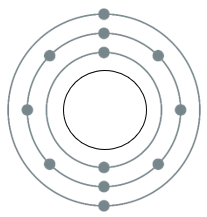d. Among the given elements, Be would be the element to which the given element resembles

Explanation:

The electronic configuration of Be is 2,2. The number of electrons present in the outermost shell is 2. Thus, Be belongs to the group 2 (alkaline earth metals). The given element also belongs to group 2.

Thus, Be resembles the given element.

Question 4.

Write down the electronic configuration of the following elements from the given atomic numbers. Answer the following question with explanation.

3Li, 14Si, 2He, 11Na, 15P

Which of these elements belong to be period 3?

Electronic configuration:

⇒ The atomic number of lithium = 3

The electronic configuration of 3Li = 2,1

⇒ The atomic number of silicon = 14

The electronic configuration of 14Si = 2,8,4

⇒ The atomic number of helium = 2

The electronic configuration of 2He = 2

⇒ The atomic number of sodium = 11

The electronic configuration of 11Na = 2,8,2

⇒ The atomic number of phosphorus = 15

The electronic configuration of 15P = 2,8,5

Among the given elements, Na(2,8,2), Si(2,8,4) and P(2,8,5) belong to group 3. Because in these elements, total three shells are present. Thus, these elements belong to group 3.

Question 5.

Write down the electronic configuration of the following elements from the given atomic numbers. Answer the following question with explanation.

1H, 7N, 20Ca, 16S, 4Be, 18Ar

Which of these elements belong to the second group?

Electronic configuration:

⇒ The atomic number of hydrogen = 1

The electronic configuration of 1H = 1

⇒ The atomic number of nitrogen = 7

The electronic configuration of 7N = 2,5

⇒ The atomic number of calcium = 2

The electronic configuration of 20Ca = 2,8,8,2

⇒ The atomic number of sulphur = 16

The electronic configuration of 16S = 2,8,6

⇒ The atomic number of beryllium = 4

The electronic configuration of 4Be = 2,2

⇒ The atomic number of argon = 18

The electronic configuration of 18Ar = 2,8,8

Among the given elements, Ca and Be belong to second group. Because both has two electrons in the outermost shell. Thus, these two elements belong to second group.

Question 6.

Write down the electronic configuration of the following elements from the given atomic numbers. Answer the following question with explanation.

7N, 6C, 8O, 5B, 13A1

Which is the most electronegative element among these?

Electronic configuration:

⇒ The atomic number of nitrogen = 7

The electronic configuration of 7N = 2,5

⇒ The atomic number of carbon = 6

The electronic configuration of 6C = 2,4

⇒ The atomic number of oxygen = 8

The electronic configuration of 8O = 2,6

⇒ The atomic number of boron = 5

The electronic configuration of 5B = 2,3

⇒ The atomic number of aluminum = 13

⇒ The electronic configuration of 13Al = 2,8,3

Among the given elements, oxygen is the most electronegative element.

Explanation: Electronegativity is the ability of an atom to attract shared pair of electrons.

⇒ Electronegativity increases as we move from left to right in a period.

⇒ Electronegativity decreases as we move down the group.

⇒ By combining these two facts, oxygen is the most electronegative element.

Note:

Electronegativity increases along a period because-

⇒ The atomic radius goes on decreasing as we move from left to right due to which the attraction between the outer electrons and nucleus increases.

Electronegativity decreases along a group because-

⇒ The atomic radius goes on increasing as we move from top to bottom due to which the attraction between the outer electrons and nucleus decreases.

Question 7.

Write down the electronic configuration of the following elements from the given atomic numbers. Answer the following question with explanation.

4Be, 6C, 8O, 5B, 13A1

Which is the most electropositive element among these?

Electronic configuration:

⇒ The atomic number of beryllium = 4

The electronic configuration of 4Be = 2,2

⇒ The atomic number of carbon = 6

The electronic configuration of 6C = 2,4

⇒ The atomic number of oxygen = 8

The electronic configuration of 8O = 2,6

⇒ The atomic number of boron = 5

The electronic configuration of 5B = 2,3

⇒ The atomic number of aluminum = 13

The electronic configuration of 13Al = 2,8,3

Among the given elements, Al has the highest electropositive character.

Explanation:

Electropositivity is the tendency of any metal element to become cation (positively charged) by losing its valence electrons.

⇒ Electropositivity decreases as we move from left to right in a period.

⇒ Electropositivity increases as we move from top to bottom in a group.

⇒ Electropositive character is shown by the metals because they have a tendency to lose electrons.

⇒ Combining all these facts, Al is the most electropositive element.

Question 8.

Write down the electronic configuration of the following elements from the given atomic numbers. Answer the following question with explanation.

11Na, 15P, 17C1, 14Si, 12Mg

Which of these has largest atoms?

Electronic configuration:

⇒ The atomic number of sodium = 11

The electronic configuration of 11Na = 2,8,2

⇒ The atomic number of phosphorus = 15

The electronic configuration of 15P = 2,8,5

⇒ The atomic number of chlorine = 17

The electronic configuration of 17Cl = 2,8,7

⇒ The atomic number of silicon = 14

The electronic configuration of 14Si = 2,8,4

⇒ The atomic number of magnesium = 12

The electronic configuration of 12Mg = 2,8,2

Among the given elements, Na is the element which has largest atoms.

Explanation:

All the given elements have total three shells present in them which means all the elements belong to the same period (period 3).

⇒ The atomic radius decreases as we move from left to right in a period.

⇒ Thus, Na has the largest atomic radius.

Question 9.

Write down the electronic configuration of the following elements from the given atomic numbers. Answer the following question with explanation.

19K, 3Li, 11Na, 4Be

Which of these atoms has smallest atomic radius?

Electronic configuration:

⇒ The atomic number of potassium = 19

The electronic configuration of 19K = 2,8,8,1

⇒ The atomic number of lithium = 3

The electronic configuration of 3Li = 2,1

⇒ The atomic number of sodium = 11

The electronic configuration of 11Na = 2,8,1

⇒ The atomic number of beryllium = 4

The electronic configuration of 4Be = 2,2

Among the given elements, Be has the smallest atomic radius.

Explanation:

⇒ Atomic radius goes on decreasing along a period.

⇒ Atomic radius goes on increasing along a group.

⇒ By combining these two facts, we can conclude that Be has the smallest atomic radius.

Question 10.

Write down the electronic configuration of the following elements from the given atomic numbers. Answer the following question with explanation.

13A1, 14Si, 11Na, 12Mg, 16S

Which of the above elements has the highest metallic character?

Electronic configuration:

⇒ The atomic number of aluminum = 13

The electronic configuration of 13Al = 2,8,3

⇒ The atomic number of silicon = 14

The electronic configuration of 14Si = 2,8,4

⇒ The atomic number of sodium = 11

The electronic configuration of 11Na = 2,8,1

⇒ The atomic number of magnesium = 12

The electronic configuration of 12Mg = 2,8,2

⇒ The atomic number of sulphur = 16

The electronic configuration of 16S = 2,8,6

Among the given elements, Na has highest metallic character (most electropositive element)

Explanation:

All the given elements have total three shells present in them which means all the elements belong to the same period (period 3).

⇒ The metallic character decreases as we move from sodium to sulphur in a period.

⇒ Hence, Na has the high tendency to loose electrons.

⇒ Thus, sodium (Na) has the highest metallic character.

Note: More easily an element loses electrons, higher will be its metallic character (electropositive character)

Question 11.

Write down the electronic configuration of the following elements from the given atomic numbers. Answer the following question with explanation.

6C, 3Li, 9F, 7N, 8O

Which of the above elements has the highest nonmetallic character?

Electronic configuration:

⇒ The atomic number of carbon = 6

The electronic configuration of 6C = 2,4

⇒ The atomic number of lithium = 3

The electronic configuration of 3Li = 2,1

⇒ The atomic number of fluorine = 9

The electronic configuration of 9F = 2,7

⇒ The atomic number of nitrogen = 7

The electronic configuration of 7N = 2,5

⇒ The atomic number of oxygen = 8

The electronic configuration of 8O = 2,6

Among the given elements, fluorine has highest non-metallic character (most electronegative element).

Explanation:

All the given elements have total two shells present in them which means all the elements belong to the same period (period 2).

⇒ The non-metallic character (electronegativity) increases as we move from lithium to fluorine in a period.

⇒ Hence, among the given elements, fluorine has the highest tendency to accept electrons.

⇒ Thus, fluorine has the highest non-metallic character.

Note: More easily an element accepts electrons, higher will be its non- metallic character (electronegativity)

Question 12.

Write the name and symbol of the element from the description.

a. The atom having the smallest size.

b. The atom having the smallest atomic mass.

c. The most electronegative atom.

d. The noble gas with the smallest atomic radius.

e. The most reactive nonmetal.

a. The atom with the smallest size is hydrogen.

The symbol of the hydrogen atom is 1H.

b. The atom with the smallest atomic mass is Hydrogen.

The symbol of the helium atom is 1H.

c. The most electronegative atom is fluorine because:

⇒ Fluorine (2,7) has the highest tendency to accept electron to achieve noble gas configuration (2,8).

⇒ As we know that more easily an atom accepts an electron, higher will be its electronegativity.

The symbol of the fluorine atom is 9F.

d. The noble gas with the smallest atomic radius is Helium.

The symbol of the hydrogen atom is 1H.

e. The most reactive non-metal is fluorine because:

⇒ Halogens are the most reactive non- metals in the periodic table.

⇒ Halogens (non-metals) have strong tendency to gain/accept electrons.

⇒ All the halogens need only one electrons to achieve noble gas configuration.

⇒ As we know that non-metallic character decreases down the group. Thus among halogens, fluorine is the most reactive non-metal.

The symbol of the fluorine atom is 9F.

Question 13.

Write short notes.

Mendeleev’s periodic law.

Mendeleev stated a periodic law. The law states that “Properties of elements are periodic function of their atomic masses.”

⇒ Mendeleev arranged the elements according to their properties and in order of increasing atomic weights.

⇒ In the Mendeleev’s periodic table, vertical columns are called groups and horizontal rows are called periods.

⇒ Atomic masses of some elements were rediscovered so as to give them proper place in the periodic table.

⇒ Mendeleev kept vacant places in the periodic table for elements not discovered till then.

Question 14.

Write short notes.

Structure of the modern periodic table.

Structure of the modern periodic table:

⇒ The modern periodic table contains horizontal periods 1 to 7.

⇒ Similarly, it contains vertical groups 1 to 18.

⇒ Atomic numbers are indicated on the upper part of the element.

⇒ Two rows are separately placed at the bottom of the periodic table. These are called lanthanide series and actinoid series.

⇒ There are 118 boxes in the periodic table.

⇒ The whole periodic table is divided into four blocks.

⇒ The left side is s-block, right side is p-block, in the middle there is d-block and the lanthanide series and actinoid series form the f-block.

⇒ The first period contains 2 elements.

⇒ The s-block contains alkali and alkaline earth metals.

⇒ The p-block contains metals, non-metals and metalloids.

⇒ The d-block contains transition metals.

Question 15.

Write short notes.

Position of isotopes in the Mendeleev’s and the modern periodic table.

Isotopes - In certain elements, some atoms have the same atomic number but different atomic mass number. Such atoms of an element are called isotopes of that element.

⇒ Isotopes were discovered long time after the Mendeleev discovered periodic table.

⇒ As we know that the isotopes have different atomic masses but same chemical properties.

⇒ Thus, the isotopes of element are placed in the same place of that of the element instead of been given different place in modern periodic table for each isotope.

Question 16.

Write scientific reasons.

Atomic radius goes on decreasing while going from left to right in a period.

Atomic radius goes on decreasing while going from left to right in a period because of the following reasons:

⇒ Within a period, the atomic number increases one by one as a result nuclear charge increases.

⇒ The outer electrons are adding in the same valence shell.

⇒ Due to increased nuclear charge, the attraction of electrons by the nucleus increases.

⇒ Therefore, the size of the atom decreases with the increase in atomic number (number of protons).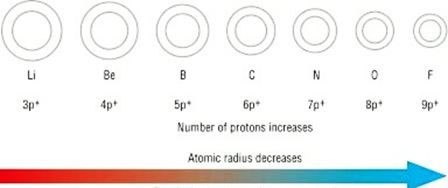Question 17.

Write scientific reasons.

Metallic character goes on decreasing while going from left to right in a period.

Metallic character goes on decreasing while going from left to right in a period because:

⇒ Metals have a tendency to lose their valence electrons to form a cation.

⇒ As we move from left to right, nuclear charge increases with increase in atomic number. This increase the force of attraction of electrons by the nucleus.

⇒ As a result, the tendency of losing electrons goes on decreasing.

⇒ Thus, metallic character decreases within a period.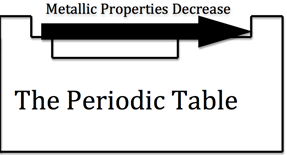Question 18.

Write scientific reasons.

Atomic radius goes on increasing down a group.

Atomic radius goes on increasing down a group because:

⇒ Down a group, the atomic number increases one by one as a result nuclear charge increases.

⇒ The outer electrons are adding in a new valence shell.

⇒ Therefore, the distance between the outermost electron (valence electron) and the nucleus is also increasing

⇒ Therefore, the size of the atom increases with increase I atomic number.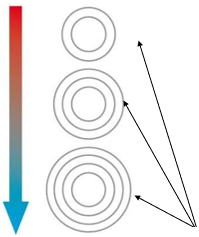Number of shells are increasing down a group

Question 19.

Write scientific reasons.

Elements belonging to the same group have the same valency.

Elements belonging to the same group have the same valency because:

⇒ Valency of an element can be determined by counting the number of electrons present in the outermost shell.

⇒ We can also determine the group number of any element by knowing its valency.

⇒ If the valency of an element is one, it means the element belongs to first group. If the valency of an element is two, this means the element belongs to second group and so on.

⇒ For example: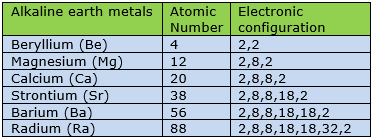As you can see in the table, all the alkaline earth metals have valency 2, thus they all belong to second group.

Question 20.

Write scientific reasons.

The third period contains only eight elements even through the electron capacity of the third shell is 18.

The third period contains only eight elements even through the electron capacity of the third shell is 18.

As we know that electron capacity of K,L,M,N shells are: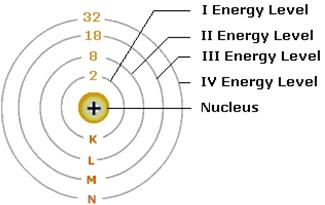But it has been observed that third shell (energy level) contains only 8 elements because:

⇒ There are many other factors which controls the filling of electrons.

⇒ The third period contains 8 elements according to the law of electron octet in which every atom aims to have eight electrons in its outer shell in order to get the noble gas configuration, any extra electrons that come go to the next shell,
*EXTRA INFORMATION
Period number is given by the last shell which an electron enters into. The electrons fill up 3s and 3p subshells (total electrons = 2+6=8, hence 8 elements), and then they start filling 4s before going back to 3d.

Question 21.

Write the names from the description.

The period with electrons in the shells K, L and M.

Third period

It is given that the electrons are present in shells K,L and M. This means there are total three shells present. Thus, third period is having elements which are electrons present in K,L and M.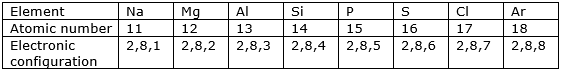Question 22.

Write the names from the description.

The group with valency zero.

Group-8 (Noble gases)

In the noble gases, there are no valence electrons present. The atoms of the noble gases have noble gas configuration as shown below in the table: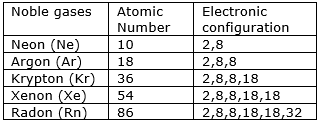Question 23.

Write the names from the description.

The family of nonmetals having valency one.

The family of nonmetals having valency one are:

⇒ Fluorine

⇒ Chlorine

⇒ Bromine

⇒ Iodine

These all non-metals need only one electron to achieve noble gas configuration.

Question 24.

Write the names from the description.

The family of metals having valency one.

The family of metals having valency one are (alkali metals):

⇒ Lithium

⇒ Sodium

⇒ Potassium

⇒ Rubidium

⇒ Cesium

⇒ Francium

Question 25.

Write the names from the description.

The family of metals having valency two.

The family of metals having valency two are (alkaline earth metals).

⇒ Beryllium

⇒ magnesium

⇒ Calcium

⇒ Strontium

⇒ Barium

Question 26.

Write the names from the description.

The metalloids in the second and third periods.

Second period and third period contain metalloids which are Boron and Silicon respectively.These are the elements having properties intermediate of metals and non-metals

Question 27.

Write the names from the description.

Nonmetals in the third period.

Answer: Non-Metals present in 3rd Period are :- Phosphorous, Chlorine and Sulfur
Question 28.

Write the names from the description.

Two elements having valency 4.

Two elements having valency four are 14Si (silicon) and 6C (carbon)

Explanation:

⇒ The atomic number of carbon = 6

The electronic configuration of 6C = 2,4

The electrons in the outermost shell = 4

Thus, its valency is 4.

⇒ The atomic number of silicon = 14

The electronic configuration of 14Si = 2,8,4

The electrons in the outermost shell = 4

Thus, its valency is 4

## PDF FILE TO YOUR EMAIL IMMEDIATELY PURCHASE NOTES & PAPER SOLUTION. @ Rs. 50/- each (GST extra)

SUBJECTS

HINDI ENTIRE PAPER SOLUTION

MARATHI PAPER SOLUTION
SSC MATHS I PAPER SOLUTION
SSC MATHS II PAPER SOLUTION
SSC SCIENCE I PAPER SOLUTION
SSC SCIENCE II PAPER SOLUTION
SSC ENGLISH PAPER SOLUTION
SSC & HSC ENGLISH WRITING SKILL
HSC ACCOUNTS NOTES
HSC OCM NOTES
HSC ECONOMICS NOTES
HSC SECRETARIAL PRACTICE NOTES

2019 Board Paper Solution

HSC ENGLISH SET A 2019 21st February, 2019

HSC ENGLISH SET B 2019 21st February, 2019

HSC ENGLISH SET C 2019 21st February, 2019

HSC ENGLISH SET D 2019 21st February, 2019

SECRETARIAL PRACTICE (S.P) 2019 25th February, 2019

HSC XII PHYSICS 2019 25th February, 2019

CHEMISTRY XII HSC SOLUTION 27th, February, 2019

OCM PAPER SOLUTION 2019 27th, February, 2019

HSC MATHS PAPER SOLUTION COMMERCE, 2nd March, 2019

HSC MATHS PAPER SOLUTION SCIENCE 2nd, March, 2019

SSC ENGLISH STD 10 5TH MARCH, 2019.

HSC XII ACCOUNTS 2019 6th March, 2019

HSC XII BIOLOGY 2019 6TH March, 2019

HSC XII ECONOMICS 9Th March 2019

SSC Maths I March 2019 Solution 10th Standard11th, March, 2019

SSC MATHS II MARCH 2019 SOLUTION 10TH STD.13th March, 2019

SSC SCIENCE I MARCH 2019 SOLUTION 10TH STD. 15th March, 2019.

SSC SCIENCE II MARCH 2019 SOLUTION 10TH STD. 18th March, 2019.

SSC SOCIAL SCIENCE I MARCH 2019 SOLUTION20th March, 2019

SSC SOCIAL SCIENCE II MARCH 2019 SOLUTION, 22nd March, 2019

XII CBSE - BOARD - MARCH - 2019 ENGLISH - QP + SOLUTIONS, 2nd March, 2019

HSC Maharashtra Board Papers 2020

(Std 12th English Medium)

HSC ECONOMICS MARCH 2020

HSC OCM MARCH 2020

HSC ACCOUNTS MARCH 2020

HSC S.P. MARCH 2020

HSC ENGLISH MARCH 2020

HSC HINDI MARCH 2020

HSC MARATHI MARCH 2020

HSC MATHS MARCH 2020

SSC Maharashtra Board Papers 2020

(Std 10th English Medium)

English MARCH 2020

HindI MARCH 2020

Hindi (Composite) MARCH 2020

Marathi MARCH 2020

Mathematics (Paper 1) MARCH 2020

Mathematics (Paper 2) MARCH 2020

Sanskrit MARCH 2020

Important-formula

THANKS